# Comparison of Audio Representation in TensorFlow

This article compares the different Audio Representations implemented in TensorFlow and TensorFlowIO.

## Load the file

`audio = tfio.audio.AudioIOTensor(filepath)audioSR = int(audio.rate.numpy())audio = audio[:]audio = tf.squeeze(audio, axis=-1)audio = tf.cast(audio, tf.float32)`

# Oscillogram

`plt.figure("Oscillo: " + filepath)plt.plot(audio.numpy())plt.show()`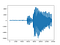Oscillogram of word “go”

Shape: (16000,)

# Spectrogram: tf.signal.stft()

`frame_step = int(audioSR * 0.008)spectrogram = tf.abs(tf.signal.stft(audio, frame_length=1024, frame_step=frame_step))plt.figure("Spect: " + filepath)plt.imshow(tf.math.log(spectrogram).numpy())plt.show()`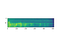Spectrogram of word “go”

Shape: (118, 513)

# Spectrogram: tfio.experimental.audio.spectrogram()

`spectrogram = tfio.experimental.audio.spectrogram(audio, nfft=1024, window=len(audio), stride=frame_step)plt.figure("Spect: " + filepath)plt.imshow(tf.math.log(spectrogram).numpy())plt.show()`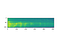Spectrogram of word “go”

Shape: (118, 513)

# MelScale: tfio.experimental.audio.melscale()

`spectrogram = tfio.experimental.audio.spectrogram(audio, nfft=1024, window=len(audio), stride=frame_step)mel = tfio.experimental.audio.melscale(spectrogram, rate=audioSR, mels=32, fmin=0, fmax=audioSR/2)plt.figure("Mel: " + filepath)plt.imshow(tf.math.log(mel).numpy())plt.show()`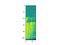MelScale of word “go”

Shape: (118, 32)

# MelDB: tfio.experimental.audio.dbscale()

`meldb = tfio.experimental.audio.dbscale(mel, top_db=60)plt.figure("MelDB: " + filepath)plt.imshow(tf.math.log(meldb).numpy())plt.show()`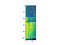MelDBScale of word “go”

Shape: (118, 32)

# Let’s compare result in machine learning

We used a word prediction system to compare each representation. Since, we only train a few files in a small number of epochs, result value are fluctuating.

Used project: https://github.com/aruno14/speechRecognition

## Spectrogram: tf.signal.stft()

Accuracy after 25 epochs: 0.59375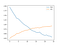Fitting history

## Spectrogram: tfio.experimental.audio.spectrogram()

Accuracy after 25 epochs: 0.6625

We obtain a little better result, however we think it is random fitting difference.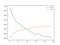Fitting history

## MelScale: tfio.experimental.audio.melscale()

Accuracy after 25 epochs: 0.7125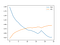Fitting history

## MelDB: tfio.experimental.audio.dbscale()

Accuracy after 25 epochs: 0.6687

# Conclusion

Regarding the input size (118, 513) VS (118, 32), and the resulting accuracy, we can say MelScaleDB is the best option for voice recognition system. Nevertheless, there are many other representations and some of them may be even better than the representation presented in this article.

However, it cost a little processing and Mel function are not widely implemented, likely in the Web Audio API.

# References

Working in computer science.

## More from Arnaud

Working in computer science.